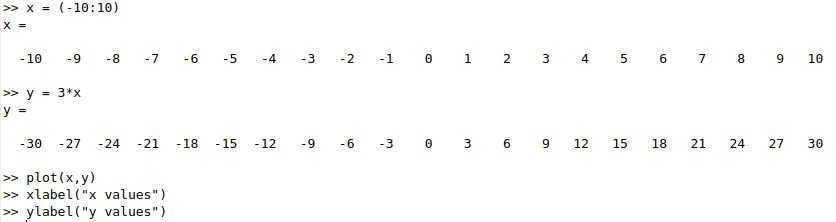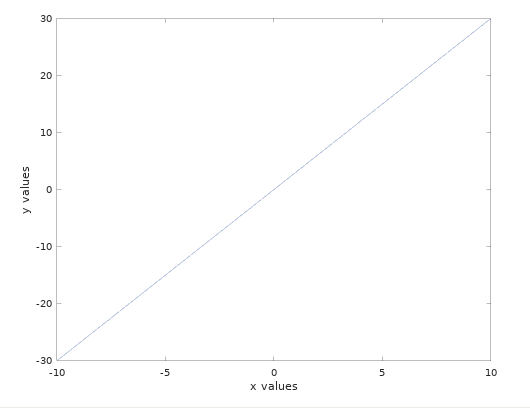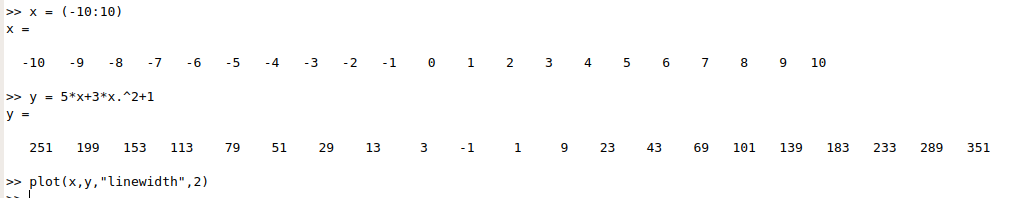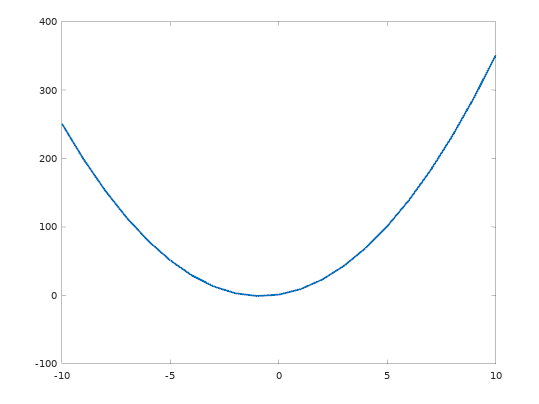# Initialize variables

## Initialize matrix with ones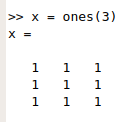## Initialize diagonal matrix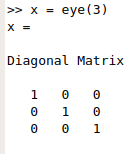## Initialize with zeros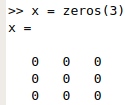## Initialize with random values: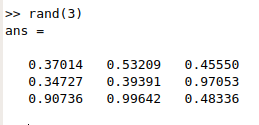## Initialize with specific value a vector or column: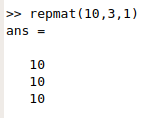## Initialize with ranges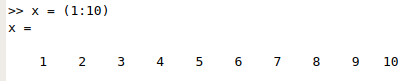## Initialize with range and steps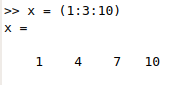Useful commands: ones(m,n), zeros(m,n), repmat(Value, m, n), eye(m,n), rand(m,n), randi(MAX value, m,n)

# Plotting

## Plotting linear equations

Let's say we want to plot this equation: y = 3x